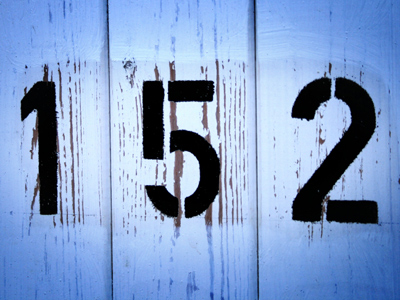152 is the next number of the sequence 112, 122, 132, 142.

# Number Sequences 1

This Math quiz is called 'Number Sequences 1' and it has been written by teachers to help you if you are studying the subject at elementary school. Playing educational quizzes is an enjoyable way to learn if you are in the 3rd, 4th or 5th grade - aged 8 to 11.

It costs only \$12.50 per month to play this quiz and over 3,500 others that help you with your school work. You can subscribe on the page at Join Us

Understanding number sequences is all about recognizing patterns in the order of numbers. We can all see that in the order 1, 2, 3, 4, 5 the numbers are increasing by 1 each time. Therefore we have found the pattern. We know that the numbers are increasing by 1 so we know that the following number will be a 6. We just need to add 1 to 5.

1.
If you add two even numbers together the answer is always what?
An odd number
An even number
A number ending in 2
A number ending in 0
2 even numbers always make an even number but an even with an odd will make an odd number
2.
If you add two odd numbers together the answer is always what?
An odd number
An even number
A number ending in 5
A number ending in 2
Even + even = even
Even + odd = odd
Odd + even = odd
Odd + odd = even
3.
Which sequence is odd?
4, 6, 8, 10, 12
30, 32, 34, 36, 38
17, 21, 25, 29, 33
12, 20, 28, 36, 44
All the numbers end in 1, 3, 5, 7 or 9 so it must be odd
4.
Can you find the rule of this sequence? 3, 13, 23, 33
The numbers increase in 2s
The numbers increase in 3s
The numbers increase in 5s
The numbers increase in 10s
Each number (except the first) is 10 higher than the one before it
5.
How do we know a number is even?
It ends in 0, 2, 4, 6 or 8
It ends in 1, 3, 5, 7 or 9
It ends in 5
It ends in 9
All the even numbers can be divided by 2 and give a whole number
6.
How do we know a number is odd?
It ends in 6
It ends in 0, 2, 4, 6 or 8
It ends in 1, 3, 5, 7 or 9
It ends in 2 or 4
If odd numbers are divided by 2 there will be a remainder or fraction left over
7.
Which is the next even number after 148?
158
150
160
152
148 + 2 = 150
8.
What are the next two numbers of the sequence? 3, 6, 9, 12
15, 18
13, 14
15, 17
15, 20
The numbers are increasing in 3s
9.
What is the next number of the sequence? 20, 120, 220, 320
300
350
400
420
The numbers are increasing by 100
10.
Can you find the rule of this sequence? 5, 10, 15, 20, 25, 30
The numbers increase in 2s
The numbers increase in 3s
The numbers increase in 5s
The numbers increase in 10s
This sequence is the 5 times table
Author:  Amanda Swift